Thursday , July 7 2022# NCERT 5th Class (CBSE) Mathematics: Area And Volume

### Area of a triangle

You can your understanding of the area of the rectangle to find the area of a triangle.

Look at the green triangle. It is exactly half of the rectangle.

The area of the triangle = Half the area of the rectangle

The formula for the rectangle is length × breadth.

Area of the rectangle = 5 cm × 4 cm = 20 sq. cm.

Areas of the triangle = 10 sq. cm.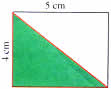Let us try to find the answer using the formula for the area of a triangle.

The base of the triangle is the same as the length of the rectangle.

The height of the triangle is the same as the breadth of the rectangle.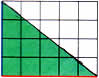Since the area of the triangle is half the area of the rectangle,

Area of the triangle = 1/2 × base × height (replacing length with base and breadth with height)

Base × Height/← Formula for area of a triangle

Area of the green triangle = 5 × 4/2 = 10 sq. cm

(a) Use the formula to find the area of triangle XYZ.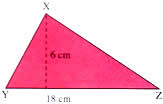Base = 18 cm

Height = 6 cm

Area = Base × Height/2

Area of ΔXYZ = 18 × 6/2 = 54 sq. cm

## VOLUME

A box of tea is much larger than matchbox. It occupies more space than a matchbox. So we can say that the box of tea has greater volume than the matchbox.

Volume is the amount of space taken up by an object.

### Measuring Volume

#### Measuring by counting

In order to measure the volume of any object, imagine it as a container that can be filled with cubes.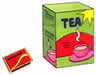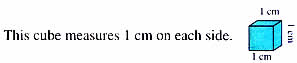Let us now find the volume of this box using centimeter cubes. Place one layer of cm cubes. Count the number of cubes you have used. There are 8 rows with 12 cubes in each row. As 12 × 8 = 96, there are 96 cubes in one layer.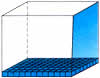## चुनौती हिमालय की 5th NCERT CBSE Hindi Rimjhim Ch 18

चुनौती हिमालय की 5th Class NCERT CBSE Hindi Book Rimjhim Chapter 18 प्रश्न: लद्दाख जम्मू-कश्मीर राज्य में …